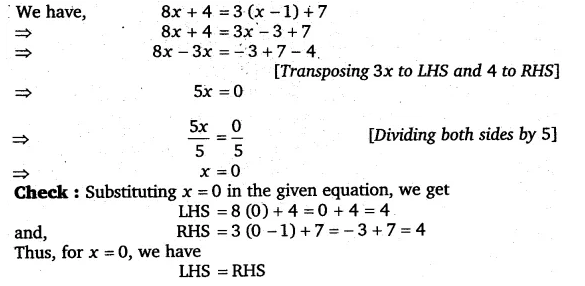# Solve the following equations and check your results: 8x + 4 = 3(x – 1) + 7

Solve the following equations and check your results:
8x + 4 = 3(x – 1) + 7